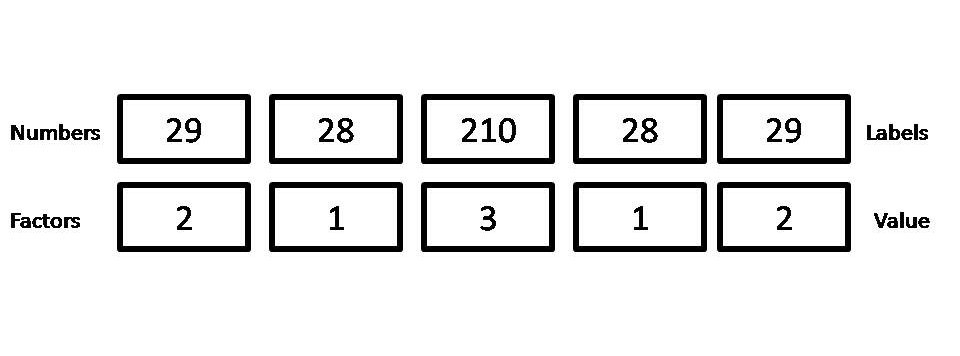# Convert Factor to Numeric and Numeric to Factor in R Programming

• Last Updated : 10 May, 2020

Factors are data structures which are implemented to categorize the data or represent categorical data and store it on multiple levels.
They can be stored as integers with a corresponding label to every unique integer. Though factors may look similar to character vectors, they are integers, and care must be taken while using them as strings.
The factor accepts only a restricted number of distinct values. It is helpful in categorizing data and storing it on multiple levels.

#### Converting Factors to Numeric Values

At times you require to explicitly change factors to either numbers or text. To achieve this, one has to use the functions `as.character()` or `as.numeric()`. There are two steps for converting factor to numeric:

Step 1: Convert the data vector into a factor. The `factor()` command is used to create and modify factors in R.

Step 2: The factor is converted into a numeric vector using `as.numeric()`.

When a factor is converted into a numeric vector, the numeric codes corresponding to the factor levels will be returned.

Example:
Take a data vector ‘V’ consisting of directions and its factor will be converted into numeric.

 `# Data Vector 'V'``V ``=` `c(``"North"``, ``"South"``, ``"East"``, ``"East"``)`` ` `# Convert vector 'V' into a factor``drn <``-` `factor(V)`` ` `# Converting a factor into a numeric vector ``as.numeric(drn)`

Output:

``` 2 3 1 1
```

Converting a Factor that is a Number:
If the factor is number, first convert it to a character vector and then to numeric. If a factor is a character then you need not convert it to a character. And if you try converting an alphabet character to numeric it will return NA.

Example:
Suppose we are taking costs of soaps of the various brands which are numbers with value s(29, 28, 210, 28, 29).

 `# Creating a Factor``soap_cost <``-` `factor(c(``29``, ``28``, ``210``, ``28``, ``29``))`` ` `# Converting Factor to numeric``as.numeric(as.character(soap_cost))`

Output:

```  29  28 210  28  29
```However, if you simply use as. numeric(), the output is a vector of the internal level representations of the factor and not the original values.

 `# Creating a Factor``soap_cost <``-` `factor(c(``29``, ``28``, ``210``, ``28``, ``29``))`` ` `# Converting Factor to Numeric``as.numeric(soap_cost)`

Output:

``` 2 1 3 1 2
```

#### Converting Numeric value to a Factor

For converting a numeric into factor we use `cut()` function. cut() divides the range of numeric vector(assume x) which is to be converted by cutting into intervals and codes its value (x) according to which interval they fall.
Level one corresponds to the leftmost, level two corresponds to the next leftmost, and so on.

Syntax:
cut.default(x, breaks, labels = NULL, include.lowest = FALSE, right = TRUE, dig.lab = 3)

where,

• When a number is given through ‘break=’ argument, the output factor is created by the division of the range of variables into that number of equal-length intervals.
• In syntax include.lowest indicates whether an ‘x[i]’ which equals the lowest (for right= TRUE) break’s value should be included. And ‘right’ in the syntax indicates whether the intervals should be open on the left and closed on the right or vice versa.
• If labels are not provided then dig.lab is used. The number of digits used in formatting the break numbers is determined through it.

Example 1:
Lets us assume an employee data set of age, salary and gender. To create a factor corresponding to age with three equally spaced levels we can write in R as follows:

 `# Creating vectors``age <``-` `c(``40``, ``49``, ``48``, ``40``, ``67``, ``52``, ``53``)  ``salary <``-` `c(``103200``, ``106200``, ``150200``, ``10606``, ``10390``, ``14070``, ``10220``)``gender <``-` `c(``"male"``, ``"male"``, ``"transgender"``, ``            ``"female"``, ``"male"``, ``"female"``, ``"transgender"``)`` ` `# Creating data frame named employee``employee<``-` `data.frame(age, salary, gender)  `` ` `# Creating a factor corresponding to age``# with three equally spaced levels``wfact ``=` `cut(employee\$age, ``3``)``table(wfact)`

Output:

```wfact
(40,49] (49,58] (58,67]
4       2       1
```

Example 2:
We will now put labels- young, medium and aged.

 `# Creating vectors``age <``-` `c(``40``, ``49``, ``48``, ``40``, ``67``, ``52``, ``53``)  ``salary <``-` `c(``103200``, ``106200``, ``150200``, ``10606``, ``10390``, ``14070``, ``10220``)``gender <``-` `c(``"male"``, ``"male"``, ``"transgender"``,``            ``"female"``, ``"male"``, ``"female"``, ``"transgender"``)`` ` `# Creating data frame named employee``employee<``-` `data.frame(age, salary, gender)  `` ` `# Creating a factor corresponding to age with labels``wfact ``=` `cut(employee\$age, ``3``, labels``=``c(``'Young'``, ``'Medium'``, ``'Aged'``))``table(wfact)`

Output:

```wfact
Young Medium   Aged
4      2      1
```

The next examples will use ‘`norm()`‘ for generating multivariate normal distributed random variants within the specified space.

There are three arguments given to rnorm():

• n: Number of random variables need to be generated
• mean: Its value is 0 by default if not mentioned
• sd: standard deviation value needs to be mentioned otherwise it is 1 by default

Syntax:

`norm(n, mean, sd)`
 `# Generating a vector with random numbers``y <``-` `rnorm(``100``)`` ` `# the output factor is created by the division``# of the range of variables into pi/3*(-3:3)``# 4 equal-length intervalsa``table(cut(y, breaks ``=` `pi``/``3``*``(``-``3``:``3``)))`

Output:

```(-3.14,-2.09] (-2.09,-1.05]     (-1.05,0]      (0,1.05]   (1.05,2.09]
1            11            26            48            10
(2.09,3.14]
4
```

The output factor is created by the division of the range of variables into 5 equal-length intervals through break argument.

 `age <``-` `c(``40``, ``49``, ``48``, ``40``, ``67``, ``52``, ``53``)  ``gender <``-` `c(``"male"``, ``"male"``, ``"transgender"``, ``"female"``, ``"male"``, ``"female"``, ``"transgender"``)`` ` `# Data frame generated from the above vectors``employee<``-` `data.frame(age, gender)  `` ` `# the output factor is created by the division ``# of the range of variables into 5 equal-length intervals``wfact ``=` `cut(employee\$age, breaks``=``5``)``table(wfact)`

Output:

```wfact
(40,45.4] (45.4,50.8] (50.8,56.2] (56.2,61.6]   (61.6,67]
2           2           2           0           1
```
 `y <``-` `rnorm(``100``)``table(cut(y, breaks ``=` `pi``/``3``*``(``-``3``:``3``), dig.lab``=``5``))`

Output:

```
(-3.1416,-2.0944] (-2.0944,-1.0472]       (-1.0472,0]        (0,1.0472]
5                13                33                28
(1.0472,2.0944]   (2.0944,3.1416]
19                 2
```

My Personal Notes arrow_drop_up